# HSSlive: Plus One & Plus Two Notes & Solutions for Kerala State Board

## AP Board Class 7 Maths Chapter 8 Exponents and Powers Ex 8.2 Textbook Solutions PDF: Download Andhra Pradesh Board STD 7th Maths Chapter 8 Exponents and Powers Ex 8.2 Book AnswersAP Board Class 7 Maths Chapter 8 Exponents and Powers Ex 8.2 Textbook Solutions PDF: Download Andhra Pradesh Board STD 7th Maths Chapter 8 Exponents and Powers Ex 8.2 Book Answers

## Andhra Pradesh State Board Class 7th Maths Chapter 8 Exponents and Powers Ex 8.2 Books Solutions

 Board AP Board Materials Textbook Solutions/Guide Format DOC/PDF Class 7th Subject Maths Chapters Maths Chapter 8 Exponents and Powers Ex 8.2 Provider Hsslive

2. Click on the Andhra Pradesh Board Class 7th Maths Chapter 8 Exponents and Powers Ex 8.2 Answers.
3. Look for your Andhra Pradesh Board STD 7th Maths Chapter 8 Exponents and Powers Ex 8.2 Textbooks PDF.
4. Now download or read the Andhra Pradesh Board Class 7th Maths Chapter 8 Exponents and Powers Ex 8.2 Textbook Solutions for PDF Free.

## AP Board Class 7th Maths Chapter 8 Exponents and Powers Ex 8.2 Textbooks Solutions with Answer PDF Download

Find below the list of all AP Board Class 7th Maths Chapter 8 Exponents and Powers Ex 8.2 Textbook Solutions for PDF’s for you to download and prepare for the upcoming exams:

Question 1.
Simplify the following by using Laws of Exponents.
(i) 37 × 38
37 × 38
We know am × an = am+n
37 × 38 = 37 + 8 = 315
∴ 37 × 38 =315

(ii) 92 × 90 × 93
92 × 90 × 93
We know ap × aq × ar = p + q + r
92 × 90 × 93 = 92 + 0 + 3 = 95
∴ 92 × 90 × 93 = 95

(iii) (28)3
(28)3
We know (am)n = amn
(28)3 = 28×3 =224
∴ (28)3 = 224

(iv) (a5)4
(a5)4
We Know (am)n = amn
(a5)4 = a5×4 = a20
(a5)4 = a20

(v) (25)4×(25)3×(25)8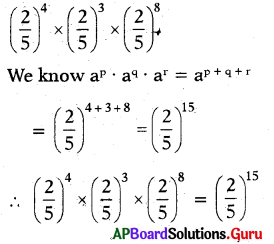(vi) 75 ÷ 78
75 ÷ 78
We know am = 1𝑎𝑛𝑚 (n>m)
75 ÷ 78 = 1785=173
∴ 75 ÷ 78 = 173

(vii) (6)9(6)5
(6)9(6)5
We Know am ÷ an = am-n(m > n)
(6)9(6)5 = (-6)9-5 = (-6)4
∴ (6)9(6)5 = (-6)4

(viii) (64 × 62) ÷ 65
(64 × 62) ÷ 65
We know atm X a = a”
= (64+2) ÷ 65
= 66 ÷ 65
We know am ÷ an = am-n (m> n)
= 66-5 = 61 = 6
∴ (64 × 62) ÷ 65 = 6

(ix) 5323
5323
We know 𝑎𝑚𝑏𝑚=(𝑎𝑏)𝑚
∴ 5323=(52)3

(x) (-3)3 × (-3)10 × (-3)7
(-3)3 × (-3)10 × (-3)7
we know ap . aq. ar = ap+q+r
(-3)3 × (-3)0 × (-3)7 = (-3)3+10+7
∴ (- 3)3 × (- 3)10 × (- 3)7 = (- 3)20

Question 2.
Simplify and express each of the following in Exponential form.
(i) (𝑎5𝑎3) × a8
(𝑎5𝑎3) × a8
We Know 𝑎𝑚𝑎𝑛 = am-n(m > n)
= (a5-3) × a8
= a2 × a8

We know am × an = am+n
= a2+8 = a10
∴ (𝑎5𝑎3) × a8 = a10

(ii) 20 + 30 – 40
20 + 30 – 40
We know a0 = 1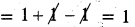∴ 20 + 30 – 40 = 1

(iii) (23 × 2)2
(23 × 2)2
We know am.an = am+n
(23 × 2)2 = (23+1)2 = (24)2

We know (am)n = am.n
= 24×2 = 28
∴(23 × 2)2 = 28

(iv) [(52)3 × 54] ÷ 57
[(52)3 × 54] ÷ 57

We know (am) = am.n
= [52×3 × 54] ÷ 57
= [56 < 54] ÷ 57

We know am .an = am+n
= [56+4] ÷ 57
= 510 ÷ 57
We know am ÷ an = am-n (m > n)
= 510-7 = 53
∴ [(52)3 × 54] ÷ 57 = 53

Question 3.
Simplify (𝑥𝑎𝑥𝑏)×(𝑥𝑏𝑥𝑐)×(𝑥𝑐𝑥𝑎)
(𝑥𝑎𝑥𝑏)×(𝑥𝑏𝑥𝑐)×(𝑥𝑐𝑥𝑎)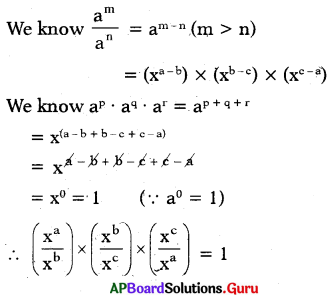Question 4.
Find the value of the following.
(i) (-1)1000
(-1)1000
(-1)1 = – 1
(-1)2 = – 1 × – 1 = + 1
(-1)3 = – 1 × -1 × -1 = – 1
(-1)4 = -1 × -1 × – 1 × – 1 = + 1
(-1)5 = -1 × -1 × -1 × -1 × -1 = -1
(-1)100 = -1 × -1 × -1 × ………… 100 times = + 1

So, (-1)odd number = -1 and
(-1)even number = + 1

Here 1000 is even number
So, (-1)1000 = + 1

(ii) (1)250
1250 = 1 × 1 × 1 ……… 250 times = 1
∴ 1250 = 1

(iii) (1)121
1121 = -1 × -1 × -1 ……..(121 times)
Here 121 is an odd number
So, (1)121 = – 1

(iv) (10000)0
(10000)0
we know a0 = 1
So, (10000)0 = 1

Question 5.
If 75 × 73x = 720 then find the value of ’x’.
Given, 75 × 73x = 720
W know am X an = am+n
75 + 3x = 720
If the bases are equal, then the powers should also be equal.
⇒ 5 + 3x = 20
⇒ 5 + 3x – 5 = 20 – 5
⇒ 3x = 15
⇒ 3𝑥3=153 = 5
∴ x = 5

Question 6.
If 10y = 10000 then 5y =?
Given, 10y = 10000
10y = 10 × 10 × 10 × 10
10y = 104
If the bases are equal, then the powers should also be equal.
⇒ y = 4
Multiply by 5 on bothsides,
5 × y = 5 × 4
∴ 5y = 20

Question 7.
If 5x = 100 then find the following values.
(i) 5x+y
Given 5x+y = 100
5x = 102
Multiply by 52
5x × 52 = 100 × 52
5x+2 = 100 × 25 (∵ am × an = am+n)
∴ 5x+2 = 2500

(ii) 5x-2
Given 5x = 100
5x = 102
Divide by 52 on both sides
5x ÷ 52 = 100 ÷ 52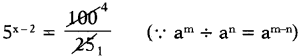∴ 5x-2 = 4

Question 8.
By what number should 34 be multiplied so that the product is 243?
Given number is 34
If we multiply 34 by ‘a’ the product is 243.
i.e. 34 × a = 243
= 3 × 3 × 3 × 3 × 3
34 × a = 35
Divide by 34 on both sides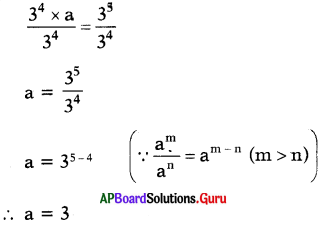If we multiply 34 by 3 we get 243.

Question 9.
Arushi computed (52)4 as 516 Has she done it correctly or not’? Justify your answer.
Given, (52)4
We know) (am)n = am.n
= 52×4 = 58
∴ (52)4 = 58
But Arushi got 516 So, (52)4 ≠ 516
Therefore Arushi did wrong.

Question 10.
Is 35 × 45 equal to 1225? If not why? Justify your answer.
Given, 35 × 45
We know am × bm = (a × b)m
So, 35 × 45 = 12 but not 125×5
= (3 × 4)5
= (12)5
35 × 45= 125
But given 35 × 45 = 1225.
So, this is wrong.
So, 35 × 45 ≠ 1225

## Andhra Pradesh Board Class 7th Maths Chapter 8 Exponents and Powers Ex 8.2 Textbooks for Exam Preparations

Andhra Pradesh Board Class 7th Maths Chapter 8 Exponents and Powers Ex 8.2 Textbook Solutions can be of great help in your Andhra Pradesh Board Class 7th Maths Chapter 8 Exponents and Powers Ex 8.2 exam preparation. The AP Board STD 7th Maths Chapter 8 Exponents and Powers Ex 8.2 Textbooks study material, used with the English medium textbooks, can help you complete the entire Class 7th Maths Chapter 8 Exponents and Powers Ex 8.2 Books State Board syllabus with maximum efficiency.

## FAQs Regarding Andhra Pradesh Board Class 7th Maths Chapter 8 Exponents and Powers Ex 8.2 Textbook Solutions

#### Can we get a Andhra Pradesh State Board Book PDF for all Classes?

Yes you can get Andhra Pradesh Board Text Book PDF for all classes using the links provided in the above article.

## Important Terms

Andhra Pradesh Board Class 7th Maths Chapter 8 Exponents and Powers Ex 8.2, AP Board Class 7th Maths Chapter 8 Exponents and Powers Ex 8.2 Textbooks, Andhra Pradesh State Board Class 7th Maths Chapter 8 Exponents and Powers Ex 8.2, Andhra Pradesh State Board Class 7th Maths Chapter 8 Exponents and Powers Ex 8.2 Textbook solutions, AP Board Class 7th Maths Chapter 8 Exponents and Powers Ex 8.2 Textbooks Solutions, Andhra Pradesh Board STD 7th Maths Chapter 8 Exponents and Powers Ex 8.2, AP Board STD 7th Maths Chapter 8 Exponents and Powers Ex 8.2 Textbooks, Andhra Pradesh State Board STD 7th Maths Chapter 8 Exponents and Powers Ex 8.2, Andhra Pradesh State Board STD 7th Maths Chapter 8 Exponents and Powers Ex 8.2 Textbook solutions, AP Board STD 7th Maths Chapter 8 Exponents and Powers Ex 8.2 Textbooks Solutions,
Share: